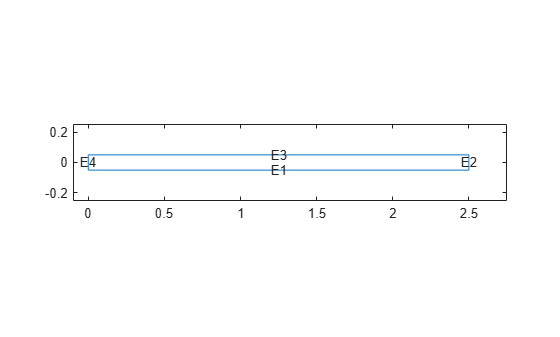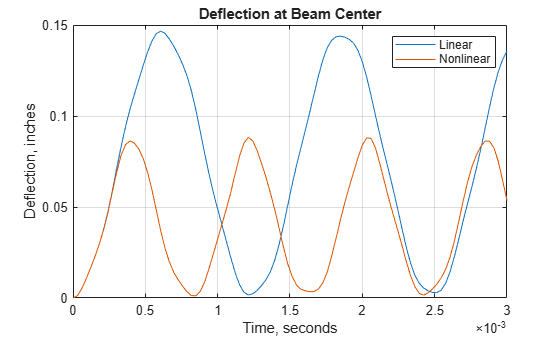Documentation

Dynamic Analysis of Clamped Beam

This example shows how to analyze the dynamic behavior of a beam clamped at both ends and loaded with a uniform pressure load.

The pressure load is suddenly applied at time equal zero and the magnitude is high enough to produce deflections on the same order as the beam thickness.

Accurately predicting this type of behavior requires a geometrically-nonlinear formulation of the elasticity equations. One of the main purposes of this example is to show how PDE Toolbox can be used to solve a problem in nonlinear elasticity. The analysis will be performed with both linear and nonlinear formulations to demonstrate the importance of the latter.

This example specifies values of parameters using the imperial system of units. You can replace them with values specified in the metric system. If you do so, ensure that you specify all values throughout the example using the same system.

Equations

This section describes the equations of geometrically nonlinear elasticity. One approach to handling the large deflections is to consider the elasticity equations in the deformed position. However, PDE Toolbox formulates the equations based on the original geometry. This motivates using a Lagrangian formulation of nonlinear elasticity where stresses, strains, and coordinates refer to the original geometry.

The Lagrangian formulation of the equilibrium equations can be written

$\rho \underset{}{\overset{¨}{u}}-\nabla \cdot \left(F\cdot S\right)=f$

where $\rho$ is the material density, $u$ is the displacement vector, $F$ is the deformation gradient, $S$ is the second Piola-Kirchoff stress tensor, and $f$ is the body force vector. This equation can also be written in the following tensor form:

$\rho {\underset{}{\overset{¨}{u}}}_{i}-\frac{\partial }{\partial {x}_{j}}\left(\left(\frac{\partial {u}_{i}}{\partial {x}_{k}}+{\delta }_{ik}\right){S}_{kj}\right)={f}_{i}$

Although large deflections are accounted for in this formulation, it is assumed that the strains remain small so that linear elastic constitutive relations apply. Also, the material is assumed to be isotropic. For the 2D plane stress case, the constitutive relations may be written in matrix form:

$\left\{\begin{array}{c}{S}_{11}\\ {S}_{22}\\ {S}_{12}\end{array}\right\}=\left[\begin{array}{ccc}{C}_{11}& {C}_{12}& \\ {C}_{12}& {C}_{22}& \\ & & 2{G}_{12}\end{array}\right]\left\{\begin{array}{c}{E}_{11}\\ {E}_{22}\\ {E}_{12}\end{array}\right\}$

where ${E}_{ij}$ is the Green-Lagrange strain tensor defined as

${E}_{ij}=\frac{1}{2}\left(\frac{\partial {u}_{i}}{\partial {x}_{j}}+\frac{\partial {u}_{j}}{\partial {x}_{i}}+\frac{\partial {u}_{k}}{\partial {x}_{i}}\frac{\partial {u}_{k}}{\partial {x}_{j}}\right)$

For an isotropic material

${C}_{11}={C}_{22}=\frac{E}{1-{\nu }^{2}}$

${C}_{12}=\frac{E\nu }{1-{\nu }^{2}}$

${G}_{12}=\frac{E}{2\left(1+\nu \right)}$

where $E$ is Young's modulus and $\nu$ is Poisson's ratio.

Readers who are interested in more details about the Lagrangian formulation for nonlinear elasticity can consult, for example, reference 1.

The equations presented above completely define the geometrically nonlinear plane stress problem. The work required to convert them to a form acceptable to PDE Toolbox is considerably simplified by using the MATLAB Symbolic Math Toolbox. Symbolic Math Toolbox can perform the necessary algebraic manipulations and output a MATLAB function defining the c-coefficient that can be passed to PDE Toolbox functions. This function, cCoefficientLagrangePlaneStress, is shown in the appendix below.

Problem Setup

Create the PDE model.

N = 2; % Two PDE in plane stress elasticity
model = createpde(N);

Define the geometry. First, specify the length and thickness of the beam,

blength = 5; % Beam length, in.
height = .1; % Thickness of the beam, in.

A drawing of the clamped beam is shown in the figure below.Since the beam geometry and loading are symmetric about the beam center(x = blength/2), the model can be simplified by considering only the right-half of the beam.

l2 = blength/2;
h2 = height/2;

Create the edges of the rectangle representing the beam with these two statements.

rect = [3 4 0 l2 l2 0 -h2 -h2  h2 h2]';
g = decsg(rect,'R1',('R1')');

The geometryFromEdges function creates a geometry object from the edges and stores it within the model.

pg = geometryFromEdges(model,g);

Plot the geometry and display the edge labels. The edge labels are needed for edge identification when applying boundary conditions.

figure
pdegplot(g,'EdgeLabels','on');
title('Geometry With Edge Labels Displayed');

Scale the plot so the labels are viewable.

axis([-.1 1.1*l2 -5*h2 5*h2]);Derive the equation coefficients using the material properties. For the linear case, the c-coefficient matrix is constant.

E = 3.0e7; % Young's modulus of the material, lbs/in^2
gnu = .3; % Poisson's ratio of the material
rho = .3/386; % Density of the material
G = E/(2.*(1+gnu));
mu = 2*G*gnu/(1-gnu);
c = [2*G+mu; 0; G;   0; G; mu; 0;  G; 0; 2*G+mu];
f = [0 0]'; % No body forces
specifyCoefficients(model, 'm', rho, 'd', 0, 'c', c, 'a', 0, 'f', f);

Apply the boundary conditions. Symmetry condition is x-displacement equal zero at left edge.

symBC = applyBoundaryCondition(model,'mixed','Edge',4,'u',0,'EquationIndex',1);

x- and y-displacements equal zero along right edge.

clampedBC = applyBoundaryCondition(model,'dirichlet','Edge',2,'u',[0 0]);

Apply a constant vertical stress along the top edge.

sigma = 2e2;
presBC = applyBoundaryCondition(model,'neumann','Edge',3,'g',[0 sigma]);

Set the zero initial displacements and velocities.

setInitialConditions(model,0,0);

Generate a mesh with approximately eight elements through the thickness of the beam.

generateMesh(model,'Hmax',height/8,'MesherVersion','R2013a');

Linear Solution

Set up the analysis timespan. Here, tfinal is the final time in the analysis.

tfinal = 3e-3;
tlist = linspace(0,tfinal,100);

Compute the time-dependent solution.

result = solvepde(model,tlist);

Interpolate the solution at the geometry center for the y-component (component 2) at all times.

xc = 1.25;
yc = 0;
u4Linear = interpolateSolution(result,xc,yc,2,1:length(tlist));

Nonlinear Solution

Specify the coefficients for the nonlinear case. The cCoefficientLagrangePlaneStress function takes the isotropic material properties and location and state structures, and returns a c-matrix for a nonlinear plane-stress analysis. Small strains are assumed; i.e. E and $\nu$ are independent of the solution. PDE Toolbox calls user-defined coefficient functions with the arguments location and state. The function cCoefficientLagrangePlaneStress expects the arguments E, gnu, location, state. c is defined below as an anonymous function to provide an interface between these two function signatures. (The function cCoefficientLagrangePlaneStress can be used with any geometric nonlinear plane stress analysis of a model made from an isotropic material.)

c  = @(location, state) cCoefficientLagrangePlaneStress(E,gnu,location,state);

specifyCoefficients(model, 'm', rho, 'd', 0, 'c', c, 'a', 0 , 'f', f);

Compute the time-dependent solution.

result = solvepde(model,tlist);

As before, interpolate the solution at the geometry center for the y-component (component 2) at all times.

u4NonLinear = interpolateSolution(result,xc,yc,2,1:length(tlist));

Plot Solutions

The figure below shows the y-deflection at the center of the beam as a function of time. The nonlinear analysis computes displacements that are substantially less than the linear analysis. This "stress stiffening" effect is also reflected in the higher oscillation frequency from the nonlinear analysis.

figure
plot(tlist,u4Linear(:),tlist,u4NonLinear(:));
legend('Linear','Nonlinear');
title 'Deflection at Beam Center'
xlabel 'Time, seconds'
ylabel 'Deflection, inches'
grid onReferences

1. Malvern, Lawrence E., Introduction to the Mechanics of a Continuous Medium, Prentice Hall, 1969.

Appendix - Nonlinear C-Coefficient Function

The function cCoefficientLagrangePlaneStress calculates the c-coefficient matrix for a large displacement Lagrangian plane stress formulation.

type cCoefficientLagrangePlaneStress
function c = cCoefficientLagrangePlaneStress(E, nu, loc, state)
%cCoefficientLagrangePlaneStress Calculate c-coefficient for nonlinear plane stress
% Calculate the c-coefficient for a geometrically nonlinear Lagrangian formulation
% of plane stress elasticity. The strain measure is the Green-Lagrange strain
% tensor. The stress is the second Piola-Kirchoff stress tensor. The material
% is assumed to be isotropic with linear behavior (Hooke's law applies).
%
% E  - Young's modulus of the linear isotropic material
% nu - Poisson's ratio for the material
% p  - matrix of point (node) locations
% t  - element connectivity matrix
% u  - current displacement vector

%    This function was generated by the Symbolic Math Toolbox version 6.0.
%    31-Jan-2014 09:50:09

% Copyright 2014-2015 The MathWorks, Inc.

ux = reshape(state.ux,2,[]);
uy = reshape(state.uy,2,[]);

dudx=ux(1,:);
dvdx=ux(2,:);
dudy=uy(1,:);
dvdy=uy(2,:);

% if(~isempty(u))
%   [ux,uy] = pdegrad(p,t,u);
%   dudx=ux(1,:); dudy=uy(1,:); dvdx=ux(2,:); dvdy=uy(2,:);
% else
%   dudx = zeros(1, size(t,2)); dudy=dudx; dvdx=dudx; dvdy=dudx;
% end

t4 = 1/(nu^2-1);
t6 = 1/(1+nu);
t7 = E*dudy.*t4*.25;
t8 = dudx+1.0;
t9 = E*dudy.*t4.*t8*.25;
t10 = dvdy+1.0;
t11 = t7+t9-E*dvdx.*t6.*t10*.25;
t12 = dvdy.*2.0;
t13 = dudx.^2;
t14 = dudy.^2;
t15 = dvdy.^2;
t16 = dvdx.^2;
t17 = E*dvdx.*t4.*(1.0./2.0);
t18 = E*dudx.*dvdx.*t4*.25;
t19 = t17+t18-E*dudy.*t6*.25-E*dudy.*dvdy.*t6.*(1.0./8.0);
t20 = E*dudy.*dvdx.*nu.*t4*.25;
t21 = t20-E*t6.*(1.0./2.0)-E*dudx.*t6*.25-E*dvdy.*t6*.25-E*dudx.*dvdy.*t6.*(1.0./8.0);
t22 = dudx.*2.0;
t23 = dvdy+2.0;
t24 = nu-1.0;
t25 = E*nu.*t4;
t26 = E*dudx.*nu.*t4.*(1.0./2.0);
t27 = E*dvdy.*nu.*t4.*(1.0./2.0);
t28 = E*dudx.*dvdy.*nu.*t4*.25;
t29 = t25+t26+t27+t28-E*dudy.*dvdx.*t6.*(1.0./8.0);
t30 = E*dudy.*t4.*t23.*(1.0./8.0);
t31 = E*dudy.*dvdy.*t4.*(1.0./8.0);
t32 = t7+t30+t31-E*dvdx.*t6.*(1.0./8.0)-E*dvdx.*t4.*t8.*t24.*(1.0./8.0);
t33 = dudy.*2.0;
t34 = dvdx.*2.0;
t35 = dudx.*dudy.*2.0;
t36 = dvdx.*dvdy;
t37 = t33+t34+t35+t36;
t38 = 1.0./t24;
t39 = E*dvdx.*t23.*t38.*(1.0./8.0);
t40 = t39-E*t6.*t37.*(1.0./8.0);
out1 = [E*t4.*(dudx.*6.0+t13.*2.0+t14+t16+4.0)*.25+E*nu.*t4.*(t12+t15)*.25;
t11;
t19;
t29;
t11;
E*t4.*(t12+t13+t14.*2.0+t15+t22+2.0)*.25+E*nu.*t4.*(t16-2.0)*.25;
t21;
t32;
t19;
t21;
E*t4.*(t12+t13+t15+t16.*2.0+t22+2.0)*.25+E*nu.*t4.*(t14-2.0)*.25;
t40;
t29;
t32;
t40;
E*t4.*(dvdy.*6.0+t14+t15.*2.0+t16+4.0)*.25+E*nu.*t4.*(t13+t22)*.25];

c = -out1([1 5 6 9 10 13 14 11 15 16], :);

end
Partial Differential Equation Toolbox DocumentationGet trial now